# R S Aggarwal Solutions for Class 10 Maths Chapter 6 Coordinate Geometry Exercise 6D

R S Aggarwal Solutions for Class 10 Maths Chapter 6 exercise 6d is equipped with all the questions provided in the textbook. Students can download free pdf by clicking on the link given below. This exercise helps students in solving problems based on Coordinate Geometry. Subject experts have crafted all the solutions using formulas for clear understanding. Download free R S Aggarwal Solutions of Class 10 pdf for Maths and score well.

## Download PDF of R S Aggarwal Solutions for Class 10 Maths Chapter 6 Coordinate Geometry Exercise 6D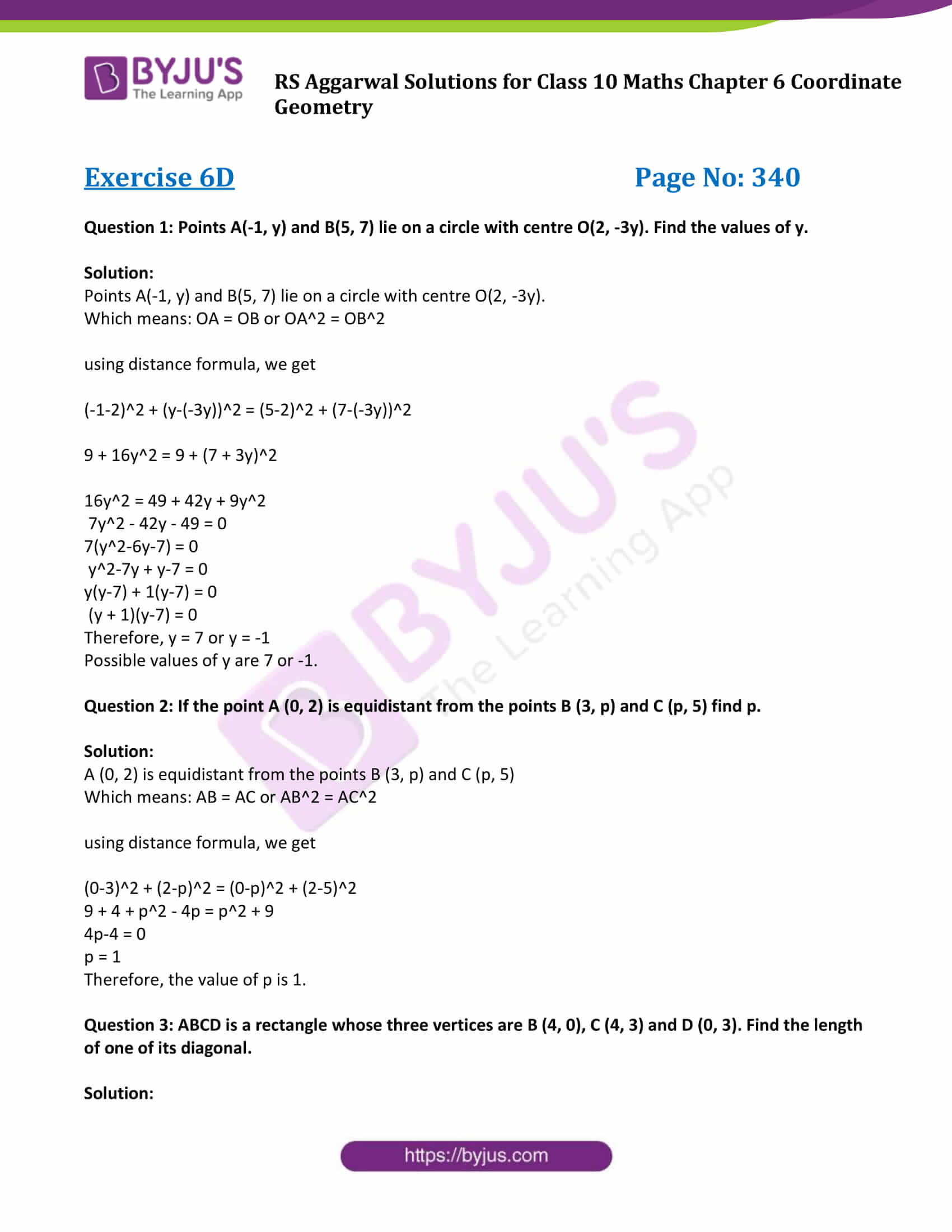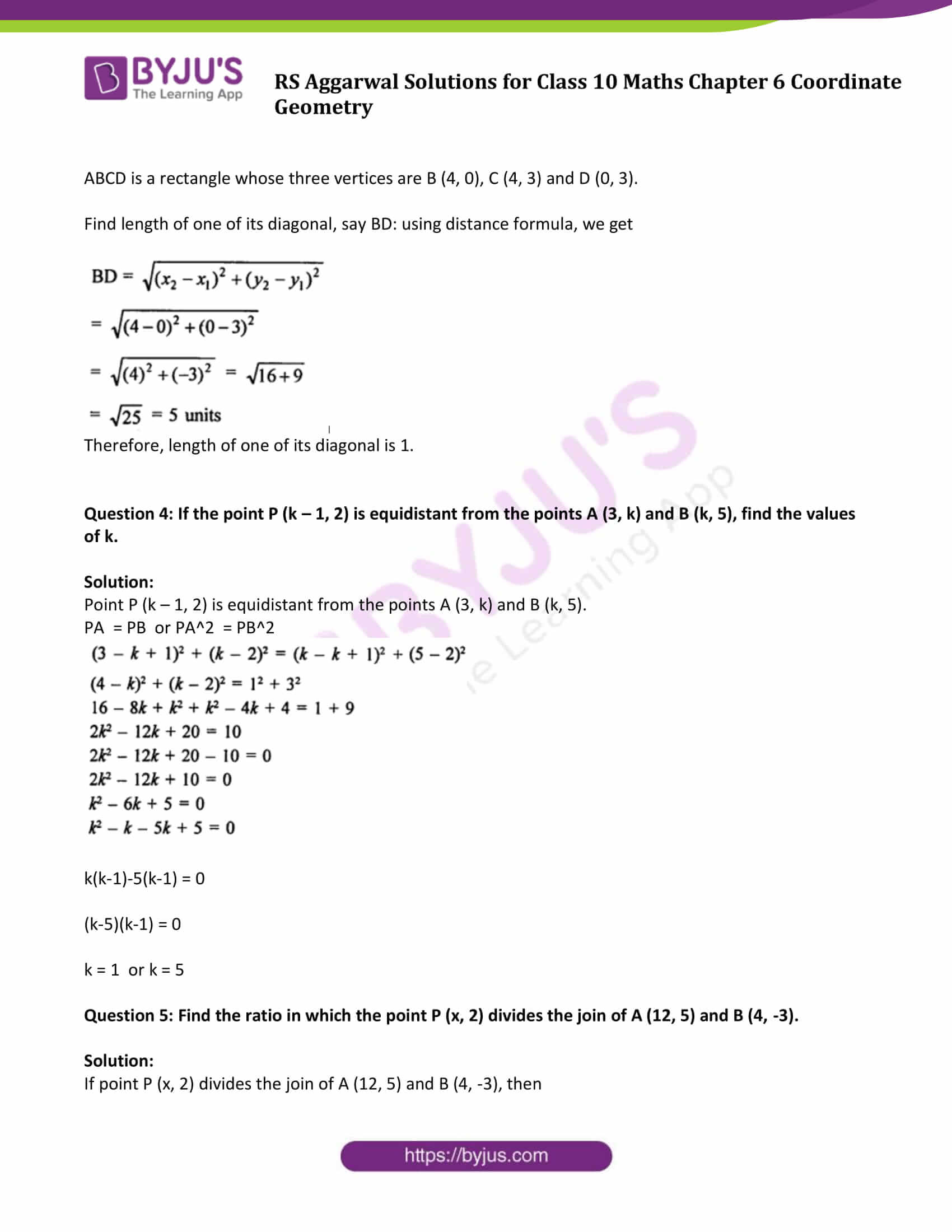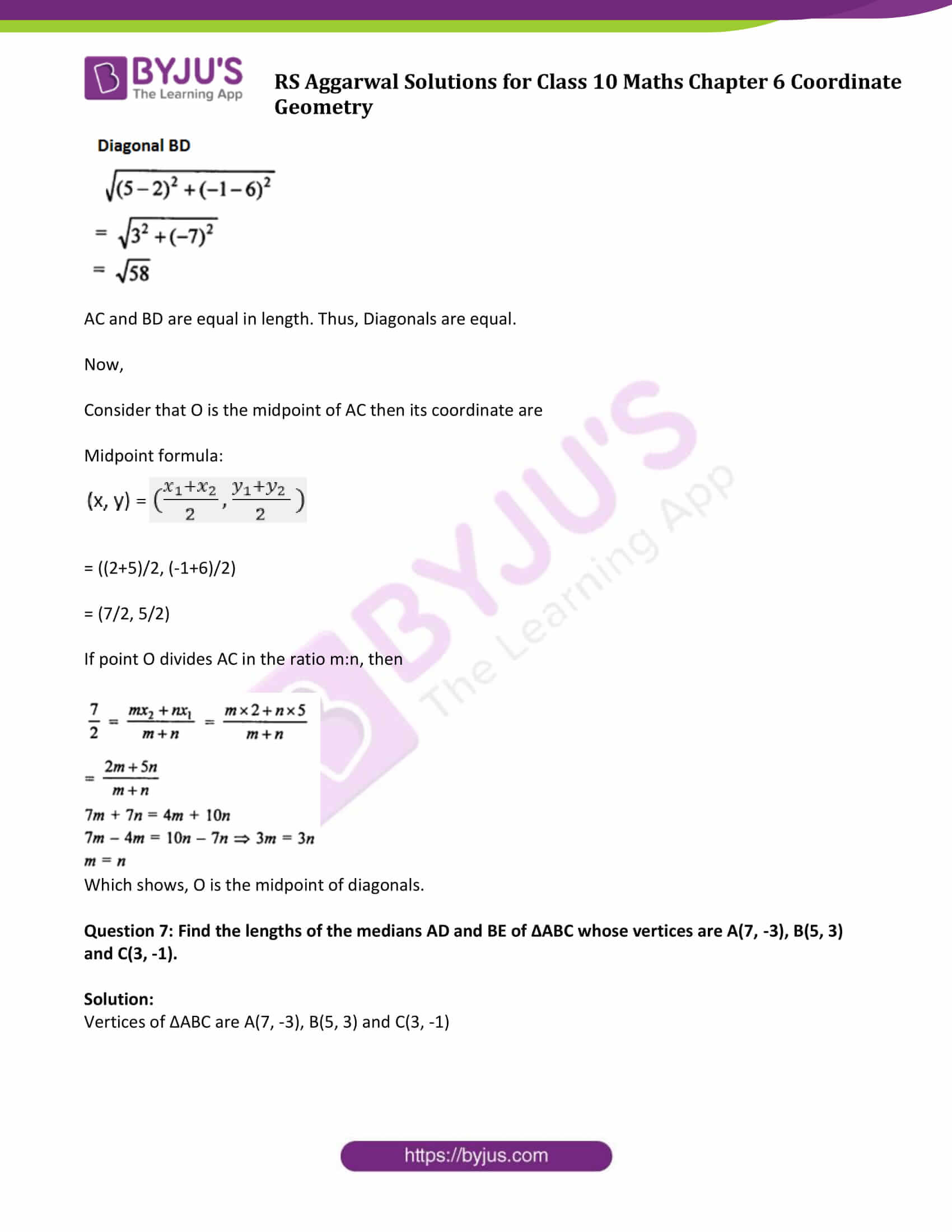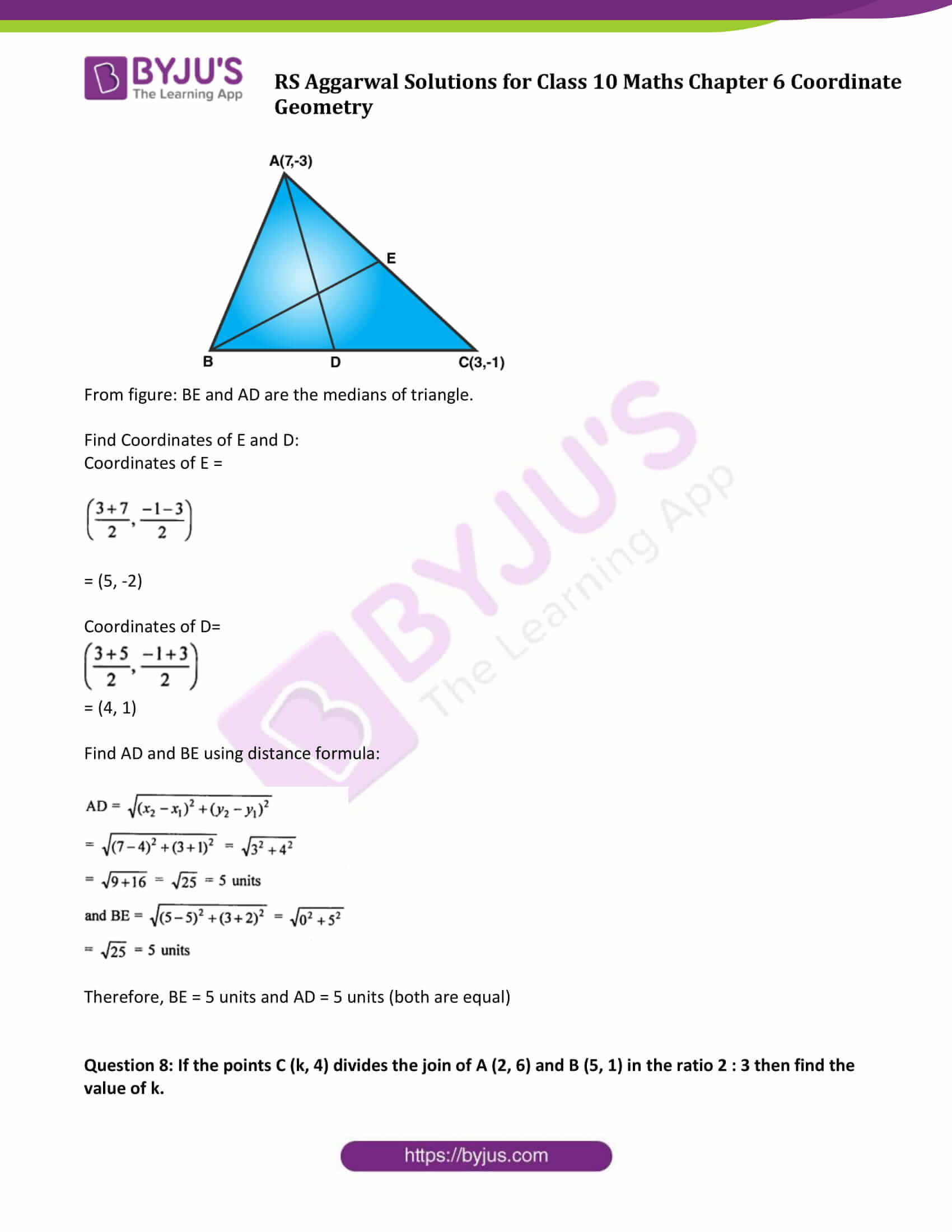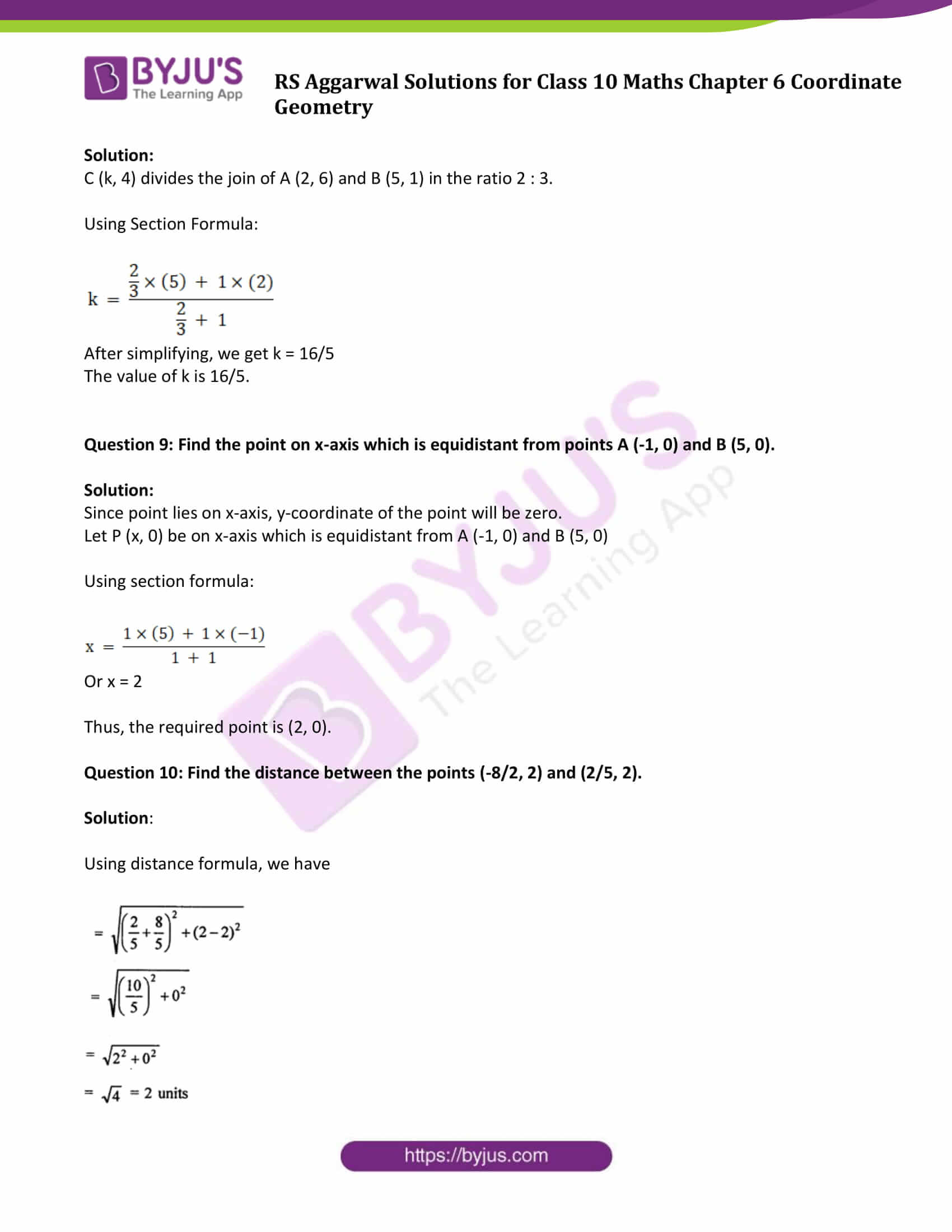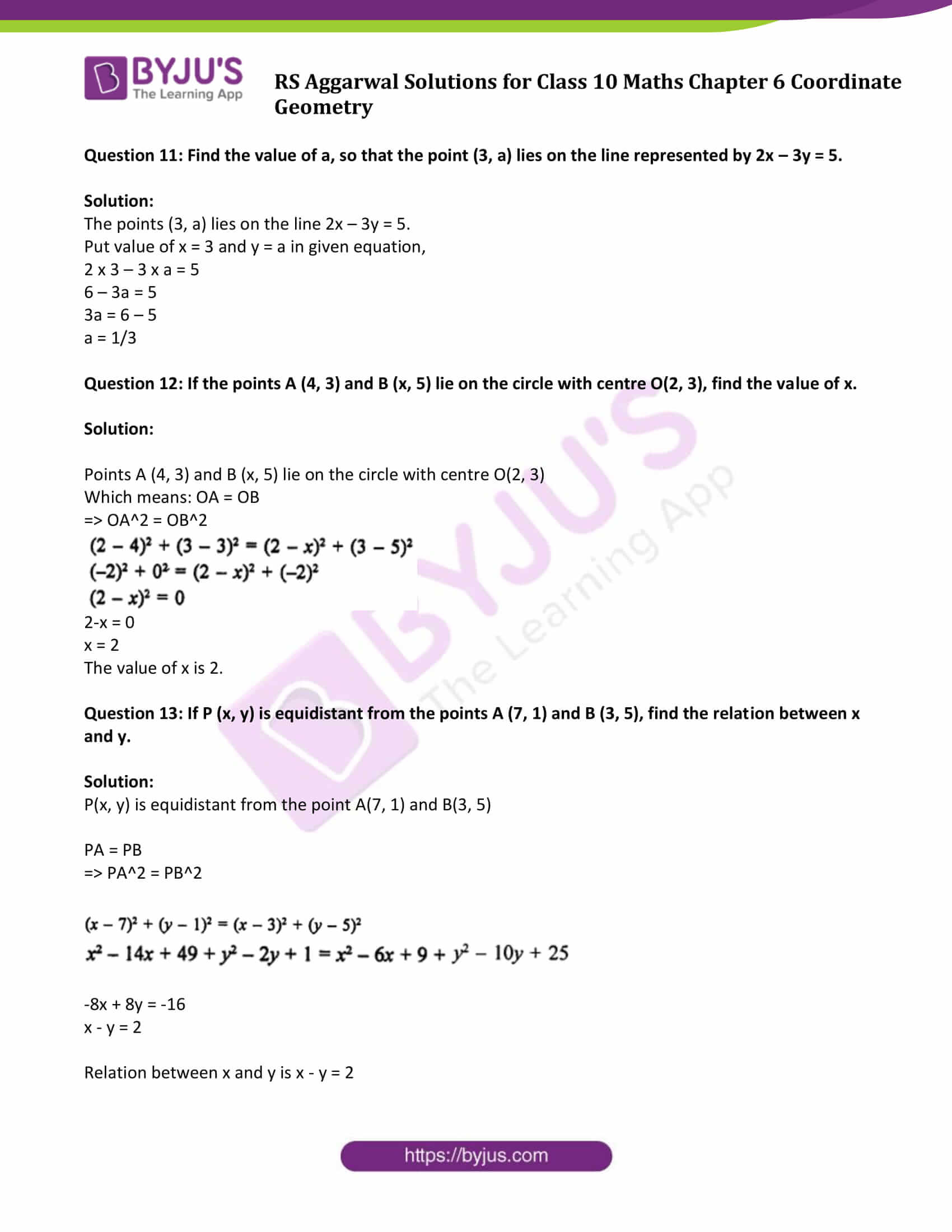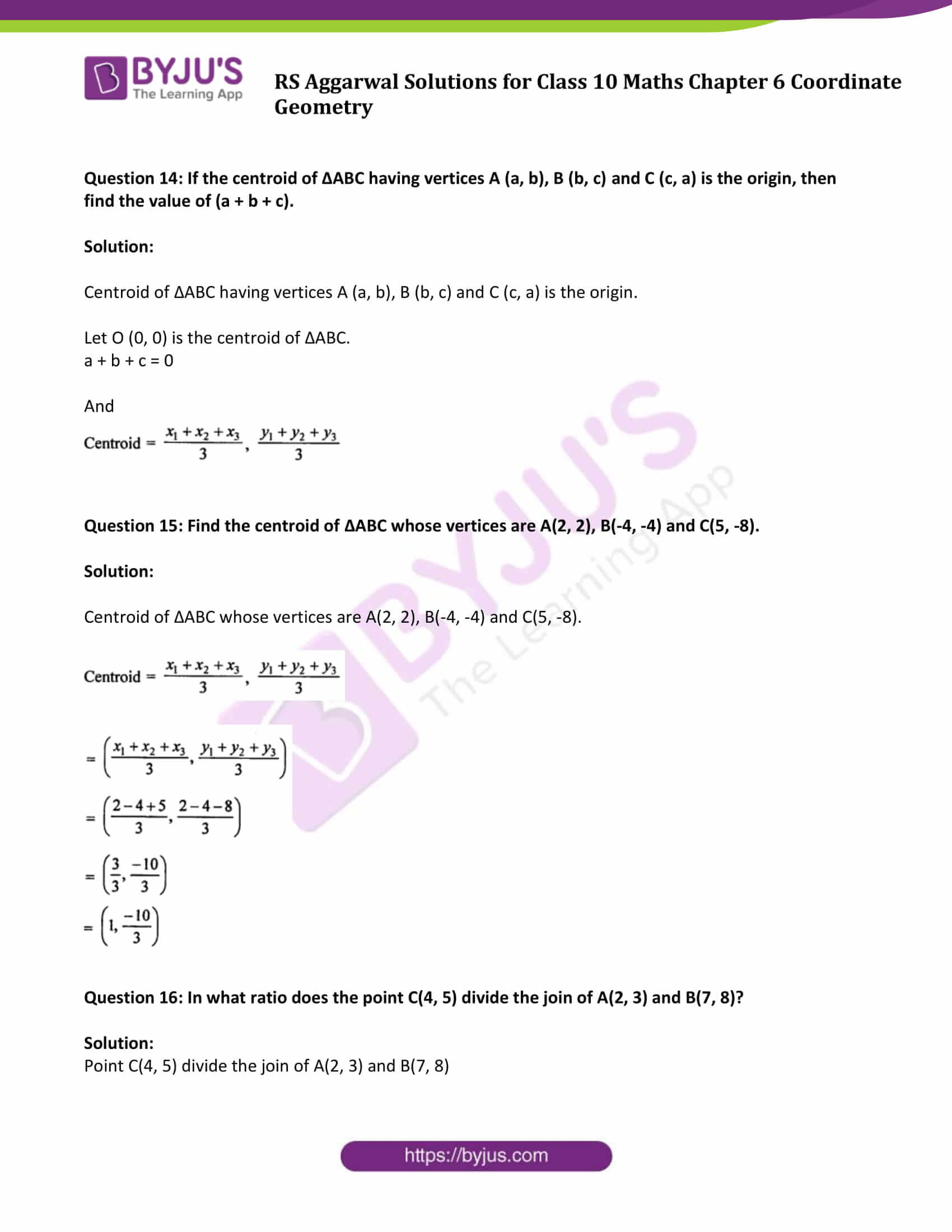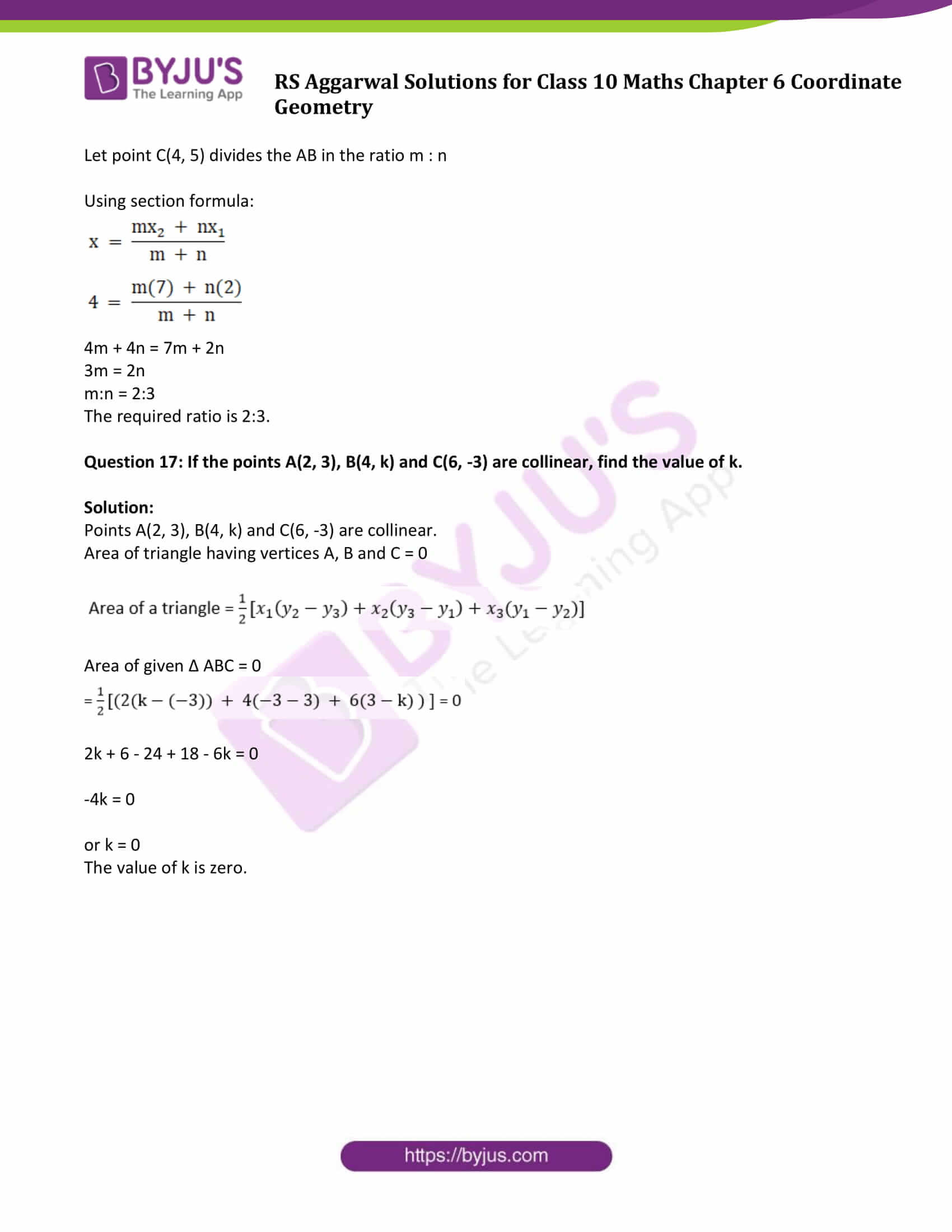## Exercise 6D Page No: 340

Question 1: Points A(-1, y) and B(5, 7) lie on a circle with centre O(2, -3y). Find the values of y.

Solution:

Points A(-1, y) and B(5, 7) lie on a circle with centre O(2, -3y).

Which means: OA = OB or OA^2 = OB^2

using distance formula, we get

(-1-2)^2 + (y-(-3y))^2 = (5-2)^2 + (7-(-3y))^2

9 + 16y^2 = 9 + (7 + 3y)^2

16y^2 = 49 + 42y + 9y^2

7y^2 – 42y – 49 = 0

7(y^2-6y-7) = 0

y^2-7y + y-7 = 0

y(y-7) + 1(y-7) = 0

(y + 1)(y-7) = 0

Therefore, y = 7 or y = -1

Possible values of y are 7 or -1.

Question 2: If the point A (0, 2) is equidistant from the points B (3, p) and C (p, 5) find p.

Solution:

A (0, 2) is equidistant from the points B (3, p) and C (p, 5)

Which means: AB = AC or AB^2 = AC^2

using distance formula, we get

(0-3)^2 + (2-p)^2 = (0-p)^2 + (2-5)^2

9 + 4 + p^2 – 4p = p^2 + 9

4p-4 = 0

p = 1

Therefore, the value of p is 1.

Question 3: ABCD is a rectangle whose three vertices are B (4, 0), C (4, 3) and D (0, 3). Find the length of one of its diagonal.

Solution:

ABCD is a rectangle whose three vertices are B (4, 0), C (4, 3) and D (0, 3).

Find length of one of its diagonal, say BD: using distance formula, we get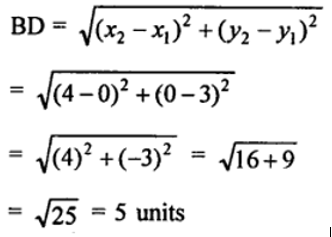Therefore, length of one of its diagonal is 1.

Question 4: If the point P (k – 1, 2) is equidistant from the points A (3, k) and B (k, 5), find the values of k.

Solution:

Point P (k – 1, 2) is equidistant from the points A (3, k) and B (k, 5).

PA = PB or PA^2 = PB^2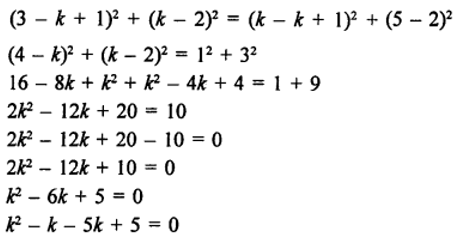k(k-1)-5(k-1) = 0

(k-5)(k-1) = 0

k = 1 or k = 5

Question 5: Find the ratio in which the point P (x, 2) divides the join of A (12, 5) and B (4, -3).

Solution:

If point P (x, 2) divides the join of A (12, 5) and B (4, -3), then

using section formula, we get

2 = (m x (-3) + n x (5)) / (m + n)

2m + 2n = -3m + 5n

5m = 3n

m/n = m:n = 3:5

The required ratio is 3:5.

Question 6: Prove that the diagonals of a rectangle ABCD with vertices A(2, -1), B(5, -1), C(5, 6) and D(2, 6) are equal and bisect each other.

Solution:

Vertices f a rectangle ABCD are A(2, -1), B(5, -1), C(5, 6) and D(2, 6)

To prove: Diagonals of the rectangle are equal and bisect each other.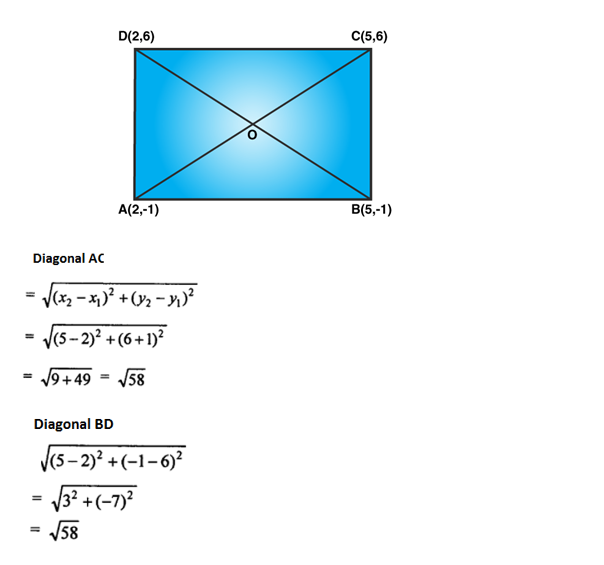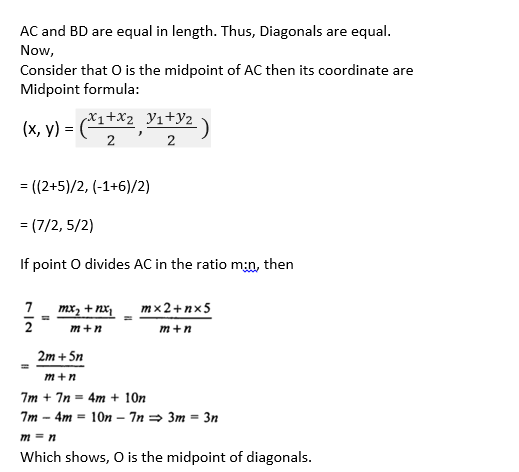Question 7: Find the lengths of the medians AD and BE of ∆ABC whose vertices are A(7, -3), B(5, 3) and C(3, -1).

Solution: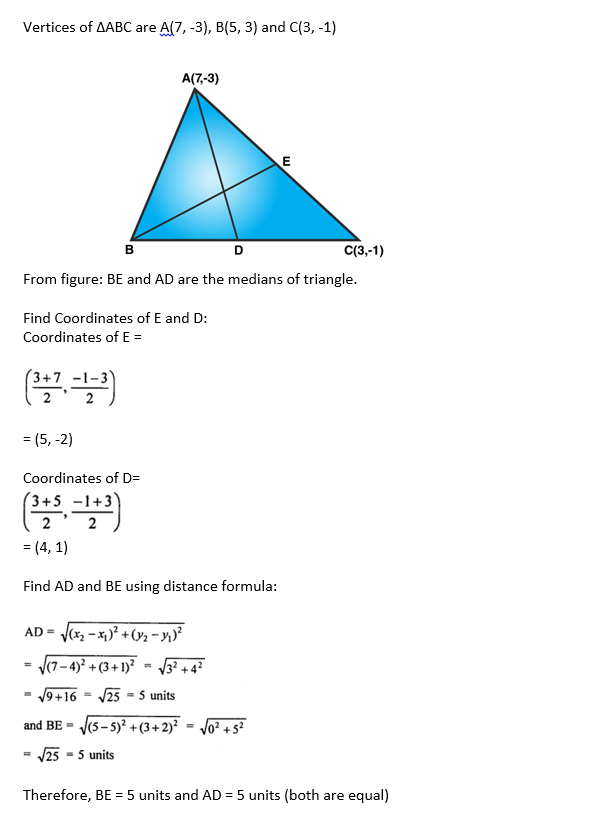Question 8: If the points C (k, 4) divides the join of A (2, 6) and B (5, 1) in the ratio 2 : 3 then find the value of k.

Solution:

C (k, 4) divides the join of A (2, 6) and B (5, 1) in the ratio 2 : 3.

Using Section Formula: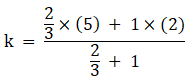After simplifying, we get k = 16/5

The value of k is 16/5.

Question 9: Find the point on x-axis which is equidistant from points A (-1, 0) and B (5, 0).

Solution:

Since point lies on x-axis, y-coordinate of the point will be zero.

Let P (x, 0) be on x-axis which is equidistant from A (-1, 0) and B (5, 0)

Using section formula: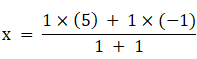Or x = 2

Thus, the required point is (2, 0).

Question 10: Find the distance between the points (-8/2, 2) and (2/5, 2).

Solution:

Using distance formula, we have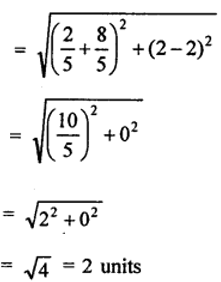Question 11: Find the value of a, so that the point (3, a) lies on the line represented by 2x – 3y = 5.

Solution:

The points (3, a) lies on the line 2x – 3y = 5.

Put value of x = 3 and y = a in given equation,

2 x 3 – 3 x a = 5

6 – 3a = 5

3a = 6 – 5

a = 1/3

Question 12: If the points A (4, 3) and B (x, 5) lie on the circle with centre O(2, 3), find the value of x.

Solution:

Points A (4, 3) and B (x, 5) lie on the circle with centre O(2, 3)

Which means: OA = OB

=> OA^2 = OB^2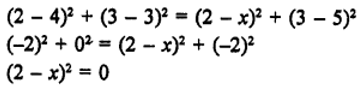2-x = 0

x = 2

The value of x is 2.

Question 13: If P (x, y) is equidistant from the points A (7, 1) and B (3, 5), find the relation between x and y.

Solution: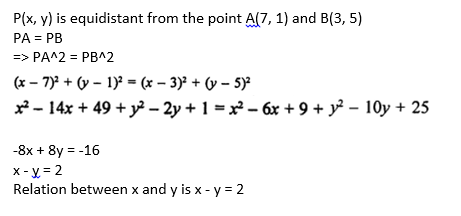Question 14: If the centroid of ∆ABC having vertices A (a, b), B (b, c) and C (c, a) is the origin, then find the value of (a + b + c).

Solution:

Centroid of ∆ABC having vertices A (a, b), B (b, c) and C (c, a) is the origin.

Let O (0, 0) is the centroid of ∆ABC.

a + b + c = 0

And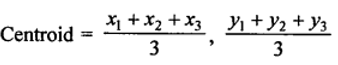Question 15: Find the centroid of ∆ABC whose vertices are A(2, 2), B(-4, -4) and C(5, -8).

Solution: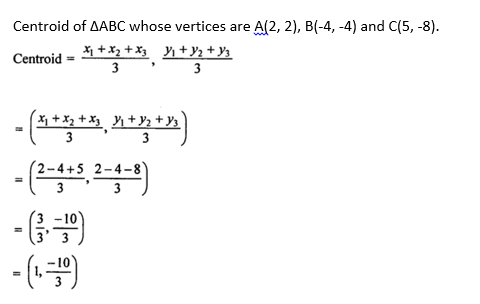Question 16: In what ratio does the point C(4, 5) divide the join of A(2, 3) and B(7, 8)?

Solution:

Point C(4, 5) divide the join of A(2, 3) and B(7, 8)

Let point C(4, 5) divides the AB in the ratio m : n

Using section formula: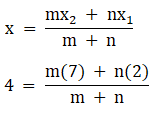4m + 4n = 7m + 2n

3m = 2n

m:n = 2:3

The required ratio is 2:3.

Question 17: If the points A(2, 3), B(4, k) and C(6, -3) are collinear, find the value of k.

Solution:

Points A(2, 3), B(4, k) and C(6, -3) are collinear.

Area of triangle having vertices A, B and C = 0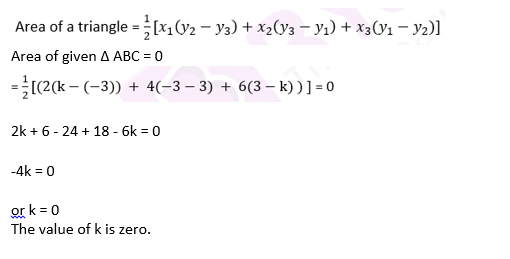### Access other exercise solutions of Class 10 Maths Chapter 6 Coordinate Geometry

Exercise 6A Solutions: 20 Questions (20 Short Answers)

Exercise 6B Solutions: 20 Questions (20 Short Answers)

Exercise 6C Solutions: 31 Questions (31 Long Answers)

## R S Aggarwal Solutions for Class 10 Maths Chapter 6 Coordinate Geometry Exercise 6D Topics

Class 10 Maths Chapter 6 Coordinate Geometry Exercise 6D is based on following topics:

• Distance between two points
• Section formula
• Midpoint Formula
• Area of a triangle